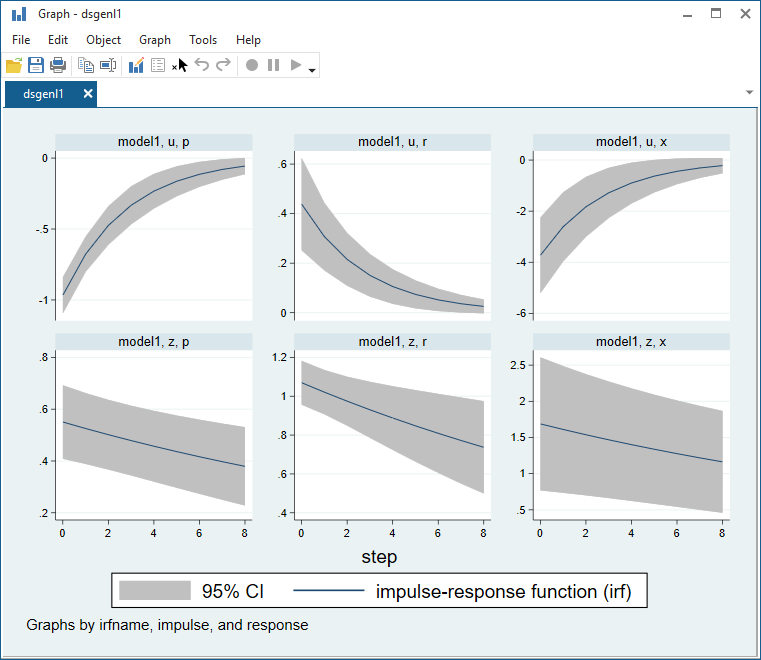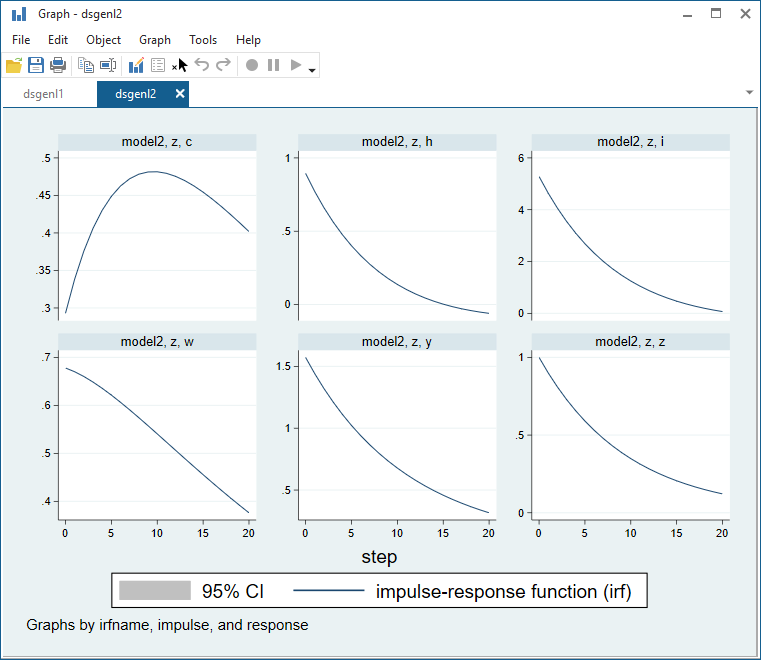»  Home »  Products »  Features »  Nonlinear DSGE

## Nonlinear DSGE

### Highlights

• Nonlinear dynamic stochastic general equilibrium models
• Model solution by first-order perturbation
• Parameter estimation
• Tables of
• Policy matrix
• Transition matrix
• Stability of model
• Model-implied covariances
• Predictions
• Expected values of dependent variables
• Expected values of unobserved state variables
• Static (one-step) predictions
• Dynamic (multistep) predictions
• RMSEs of predictions
See all features

Dynamic stochastic general equilibrium (DSGE) models are used in macroeconomics to describe the structure of the economy. These models consist of systems of equations that are derived from economic theory. In these models, expectations play an important role in determining the values of variables today. What distinguishes the DSGE model from other time-series models is its close connection to theory and the appearance of expectations in the model.

Macroeconomists use DSGEs to evaluate the impact of policy on outcomes such as output growth, inflation, and interest rates. A DSGE model can nest multiple theories. Researchers can then use estimated parameter values to determine which theory better fits the data.

Stata's dsgenl command estimates the parameters of DSGEs that are nonlinear in both the parameters and variables by using a first-order approximation to the model's equations at the steady state.

## Let's see it work

A DSGE model might propose relationships among economic variables like inflation, the interest rate, and the output gap. Some of these variables are observable, such as inflation. Others are not, such as the output gap.

These variables are linked by a system of equations. Different theories propose different forms of the equations. Parameter estimates can help us discriminate among theories.

Some of these equations will feature forward-looking elements so that expectations of future economic developments will affect variables in the current period.

Finally, the model will be driven by shocks, and these shocks will feed into the model through state variables.

We have such a model, a small one, that looks like this:

. webuse usmacro2

. dsgenl (              1 = {beta}*(x/F.x)*(r/(F.p*z)))
(1/{phi} + (p-1) = {phi}*x + {beta}*(F.p-1)  )
(       {beta}*r = p^(1/{beta})*u	       )
(        ln(F.u) = {rhou}*ln(u)	       )
(        ln(F.z) = {rhoz}*ln(z)	       )
, observed(r p) unobserved(x) exostate(z u)



Although the model is small, it still has a lot of structure.

Each line except the last defines an equation. In the equations, names enclosed in braces such as {phi} are parameters to be estimated. {phi} will turn out to be the price-adjustment parameter.

The first equation is the relationship between the output gap x and its determinants, namely

• its future value F.x,
• the nominal interest rate r,
• future inflation F.p,
• and a state variable z that captures the remaining unmodeled factors.

The second equation is the pricing equation. It links inflation to future inflation and the output gap.

The third equation is the interest-rate equation that links the interest rate to inflation and other factors u. Think of u as capturing surprise movements in the interest rate due to monetary policy.

The first three equations will be recognizable to specialists as the output gap Euler equation, a version of the New Keynesian Phillips Curve, and a version of the Taylor rule.

The final two equations specify that the state variables z and u follow autoregressive processes, albeit in logarithms.

The last line contains the options. We tell dsgenl which roles each variable plays in the model. The options are observed(r p), unobserved(x), and exostate(z u). exostate() stands for exogenous state variables. endostate() variables are also a possibility, but this model does not have any.

We estimate the parameters of the model—the terms in curly braces.

. webuse usmacro2

. dsgenl (              1 = {beta}*(x/F.x)*(r/(F.p*z)))
(1/{phi} + (p-1) = {phi}*x + {beta}*(F.p-1)  )
(       {beta}*r = p^(1/{beta})*u	       )
(        ln(F.u) = {rhou}*ln(u)	       )
(        ln(F.z) = {rhoz}*ln(z)	       )
, observed(r p) unobserved(x) exostate(z u)

Solving at initial parameter vector ...
Checking identification ...

First-order DSGE model

Sample: 1955q1 thru 2015q4                                         Number of obs = 244
Log likelihood = -753.57131

OIM
Coef.   Std. Err.      z     P>|z|      [95% Conf. Interval]

/structural
beta      .5146675   .0783486     6.57   0.000     .3611071    .6682278
phi      6.027524   1.722547     3.50   0.000     2.651394    9.403655
rhou      .7005484    .045259    15.48   0.000     .6118425    .7892543
rhoz      .9545256   .0186427    51.20   0.000     .9179866    .9910645

sd(e.z)     .6211211   .1015034                       .422178    .8200642
sd(e.u)     2.318202   .3047426                      1.720918    2.915487



Parameters beta and phi can be interpreted in terms of economic theory. Let's start with phi. It is the price adjustment parameter that is linked to the slope of the Phillips curve. Some theories predict it will be zero, and others predict that it will be positive.

Concerning beta, 1/beta is the inflation response coefficient in the Taylor rule. The point estimate for 1/beta is about 2, in line with results from the literature.

An impulse–response function traces out how shocks to state variables propagate through the model. We mentioned that state variable u can represent the stance of monetary policy. Let's see how an unexpected tightening of the policy, say, a surprise increase in interest rates, influences other variables.

. irf set dsgeirf.irf
(file dsgeirf.irf created)
(file dsgeirf.irf now active)

. irf create model1
(file dsgeirf.irf updated)

. irf graph irf, impulse(z u) response(x p r) byopts(yrescale)We find that monetary tightening leads interest rates to rise. As a result, inflation falls and the output gap falls. Thus, the model predicts a recession in response to a surprise increase in the interest rate.

## Let's see it work again

dsgenl has lots of postestimation features. We can use them after estimation to view the steady state vector, the policy matrix, and the state-transition matrix.

Consider a variant on the neoclassical growth model. It describes relationships among GDP, the major components of GDP like consumption and investment, and factors of production like labor, capital, and productivity.

The model will be

. dsgenl  (        1/c = {beta}*(1/F.c)*(1+F.r-{delta}))
(          r = {alpha}*y/k                    )
(          y = z*k^({alpha})*h^(1-{alpha})	)
(          w = (1-{alpha})*(y/h)		)
({chi}/(1-h) = w/c				)
(          y = c + i				)
(        F.k = i + (1-{delta})*k		)
(    ln(F.z) = {rho}*ln(z)			)
, observed(y) unobserved(c i w h r)
endostate(k) exostate(z)


The above command would fit the model, but that is not what we are going to do. We are going to solve the model at values of the parameters, namely values common in the literature, which are

 {beta} = 0.99
{alpha} = 0.36
{delta} = 0.025
{chi} = 2
{rho} = 0.9


To do that, we will create a parameter vector in Stata. The vector will contain values. The order in which we specify the values will not matter, because Stata vectors have row and column names associated with them, and we set the appropriate column names.

Here we create the parameter vector.


. matrix param          = (0.99, 0.36, 0.025, 2, 0.9)

. matrix colnames param = beta   alpha delta chi rho



Then we are going to issue the above, adding three additional options.

 option purpose from(param) use these parameters solve solve the model using the parameters noidencheck do not check for identification

Put it all together, and here is the result.

. matrix param          = (0.99, 0.36, 0.025, 2, 0.9)

. matrix colnames param = beta   alpha delta chi rho

. dsgenl  (        1/c = {beta}*(1/F.c)*(1+F.r-{delta}) )
(          r = {alpha}*y/k			 )
(          y = z*k^({alpha})*h^(1-{alpha})	 )
(          w = (1-{alpha})*(y/h)		 )
({chi}/(1-h) = w/c				 )
(          y = c + i				 )
(        F.k = i + (1-{delta})*k		 )
(    ln(F.z) = {rho}*ln(z)			 )
, observed(y) unobserved(c i w h r)
endostate(k) exostate(z)
solve noidencheck from(param)
Solving at initial parameter vector ...

First-order DSGE model

Sample: 1955q1 thru 2015q4                                   Number of obs = 244
Log likelihood =  -1078.509

OIM
y      Coef.   Std. Err.      z    P>|z|     [95% Conf. Interval]

/structural
beta        .99          .        .       .            .           .
delta       .025          .        .       .            .           .
alpha        .36          .        .       .            .           .
chi          2          .        .       .            .           .
rho         .9          .        .       .            .           .

sd(e.z)         1          .                             .           .

Note: Skipped identification check.
Note: Model solved at specified parameters; maximization options ignored.


Standard errors and related statistics are not reported because we did not estimate the model. We solved for a given parameter vector.

Once the model has been solved, we can use estat commands to explore the model's properties. Let's explore the steady state, policy and state-transition matrices, and impulse–response functions.

In a DSGE model, in the absence of shocks, there is a point to which the model settles down to its long-run steady state. estat steady displays that location



Delta-method
Coef.   Std. Err.      z    P>|z|     [95% Conf. Interval]

k        11.42967          .        .       .            .           .
z               1          .        .       .            .           .
c        .8286829          .        .       .            .           .
i        .2857417          .        .       .            .           .
w        2.370598          .        .       .            .           .
h        .3008658          .        .       .            .           .
r         .035101          .        .       .            .           .
y        1.114425          .        .       .            .           .

Note: Standard errors reported as missing for constrained steady state
values.


If you were interested in this model (some people are), the values just reported might interest you. Or ratios of the values would be of interest, such as the consumption-to-output ratio of roughly 75%.

The policy and state-transition matrices are approximations to the model's policy and state-transition functions. They display the impact effects of a change in a state variable on the control variables and on the expected future values of the state variables.

Here is the policy matrix. We specify option compact to report results more densely.


. estat policy, compact

Policy matrix

k          z

c     .5659819   .2928678
i    -.8879074   5.284044
w     .4538247   .6779014
h    -.2606242   .8947184
r    -.8067995    1.57262
y     .1932005    1.57262



These values reported are percentage deviations from steady state.

The last row of the second column is the impact of z on y, or productivity on output. 1.57262 means that when productivity rises by one percent, output y rises by 1.57 percent.

Here is the state-transition matrix:


. estat transition, compact

Transition matrix of state variables

k          z

k
F1.   .9528023   .1321011

z
F1.          0         .9



The transition matrix reports what is expected to happen if a state variable rises by 1% today. For instance, if z (productivity) rises, the next period's k (capital expenditures) are expected to rise by 0.13%.

Finally, we view dynamic effects by graphing the impulse–response function. The first command calculates the impulse–response function for 20 periods in the future, and the second command graphs it.

. irf create model2, step(20)
(file dsgeirf.irf updated)

. irf graph irf, impulse(z) response(y c i h w z) irf(model2) byopts(yrescale)The vertical distances are percentage deviations from steady-state values. The impulse is to z (productivity). We see the response of z itself in the bottom-right panel. After a shock, productivity declines smoothly back to its steady-state value.

Consumption's (c) response is interesting. Given an impulse, consumption rises for multiple periods before declining back to the steady state.